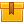# Measurement (A4307)

## Prerequisites

This lesson is designed to build on the skills presented in the fifth lesson of this program Introduction to Algebra.

## Description

This lesson reviews the concepts and basic procedures of recognizing, converting, and solving problems using the English and the metric measurement systems. It introduces strategies that participants can apply to estimate metric and English equivalents as well as solving measurement problems with or without a calculator. In addition, participants learn the process of setting up and solving measurement problems with multiple conversions using unit analysis.

## Objectives

Recognize and use common English measurement units for length, area, and volume. Recognize and use common English measurement units for weight, temperature, and time. Convert from one unit to another in the English measurement system. Convert from one unit to another in the English system using ratio. Convert units using a calculator. Explain the organization of the metric system. Recognize and use common metric units for length, area, volume, weight, and temperature. Convert from one unit to another in the metric system. Estimate metric/English equivalents. Convert length, weight, and capacity. Convert temperature between Fahrenheit and Centigrade. Multiply, divide, add, and subtract measurement units. Properly set up and solve measurement problems with multiple conversions using unit analysis. Solve measurement problems with or without a calculator.

## ContentThis lesson reviews the concepts and basic procedures of recognizing,
converting, and solving problems using the English and the metric
measurement systems. It introduces strategies that participants can
apply to estimate metric and English equivalents as well as solving
measurement problems with or without a calculator. In addition,
participants learn the process of setting up and solving measurement
problems with multiple conversions using unit analysis.

•Measurement

0
0 Reviews